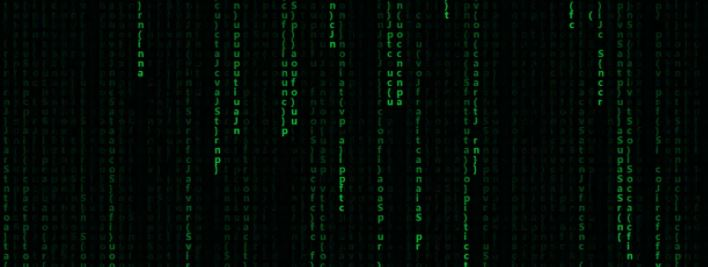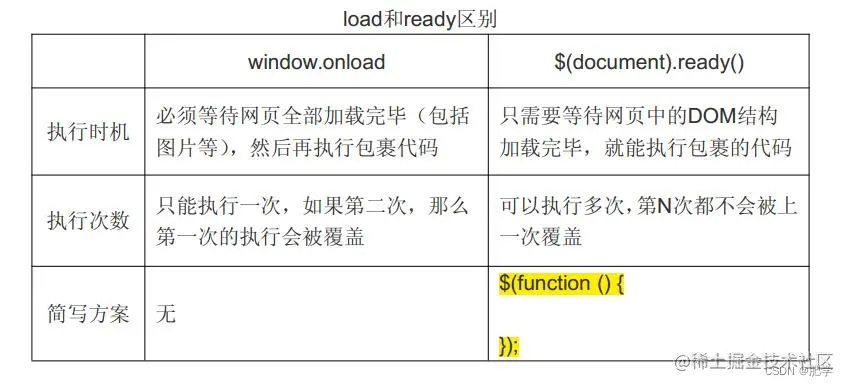﻿ 利用JS打造黑客代码雨效果_javascript技巧_脚本之家
javascript技巧# 利用JS打造黑客代码雨效果

## 演示## 技术栈

js实战我们也写过很多了，其中每次几乎都用到画布，大家知道它的重要性了吧。今天依旧用到它了。不过我们讲过它的用法就不多说了。 这次我们说一下`window.onload`

window.onload() 方法用于在网页加载完毕后立刻执行的操作，即当 HTML 文档加载完毕后，立刻执行某个方法。

```window.onload = funcRef;
```

```window.onload = function () {};    // JavaScript
\$(document).ready(function () {}); // jQuery```## 源码

### 画布

` <canvas id="mom" style="background:#111"></canvas>`

### js样式设置

```  window.onload = function(){
//获取画布对象
var canvas = document.getElementById("mom");
//获取画布的上下文
//getContext() 方法返回一个用于在画布上绘图的环境。
var context =canvas.getContext("2d");
//获取浏览器屏幕的宽度和高度
var W = window.innerWidth;
var H = window.innerHeight;
//设置canvas的宽度和高度
canvas.width = W;
canvas.height = H;
//每个文字的字体大小
var fontSize = 16;
//计算列
var colunms = Math.floor(W /fontSize);
//记录每列文字的y轴坐标
var drops = [];
//给每一个文字初始化一个起始点的位置
//计算每一个文字所谓坐标 存储y轴的坐标
for(var i=0;i<colunms;i++){
drops.push(0);
}
//运动的文字
var str ="JavaScript function(){}";
//4:fillText(str,x,y);原理就是去更改y的坐标位置
//绘画的函数
function draw(){
context.fillStyle = "rgba(0,0,0,0.05)";
//fillRect() 方法绘制“已填色”的矩形。默认的填充颜色是黑色。
context.fillRect(0,0,W,H);
//给字体设置样式
context.font = "700 "+fontSize+"px  微软雅黑";
//给字体添加颜色
context.fillStyle ="#00cc33";//可以rgb,hsl, 标准色，十六进制颜色
//写入画布中
for(var i=0;i<colunms;i++){
var index = Math.floor(Math.random() * str.length);//设置文字出发时间随机 Math.floor(Math.random()*str.length)让数组里面的文字索引随机出现
var x = i*fontSize;
var y = drops[i] *fontSize;//也让y轴方向也向下掉一个文字的距离
context.fillText(str[index],x,y);
// //如果要改变时间，肯定就是改变每次他的起点
if(y >= canvas.height && Math.random()>0.99){
drops[i] = 0;
}
drops[i]++;//让数组里面的值每次加一，用于上面的y轴下掉
}
};
//随机颜色
function randColor(){
var r = Math.floor(Math.random() * 256);
var g = Math.floor(Math.random() * 256);
var b = Math.floor(Math.random() * 256);
return "rgb("+r+","+g+","+b+")";
}
draw();
setInterval(draw,20);
};```